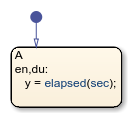# elapsed

Time since state became active

## Syntax

``elapsed(sec)``
``et``

## Description

example

````elapsed(sec)` returns the length of time that has elapsed since the associated state became active.```

example

````et` is an alternative way to execute `elapsed(sec)`.```

Note

The expressions `elapsed(sec)` and `et` are equivalent to `temporalCount(sec)`.

## Examples

expand all

Store the number of seconds since the state became active.

```en,du: y = elapsed(sec);```When the chart processes a broadcast of the event `E`, transition out of the associated state and display the elapsed time since the state became active.

`E{disp(et);}`## Tips

• In state and transition actions, you can use quotation marks to enclose the keyword `'sec'`. For example, `elapsed('sec')` is equivalent to `elapsed(sec)`.

• The Stateflow® chart resets the counter used by the `elapsed` operator each time the associated state reactivates.

• The timing for absolute-time temporal logic operators depends on the type of Stateflow chart:

• Charts in a Simulink® model define temporal logic in terms of simulation time.

• Standalone charts in MATLAB® define temporal logic in terms of wall-clock time.

The difference in timing can affect the behavior of a chart. For example, suppose that this chart is executing the `entry` action of state `A`.• In a Simulink model, the function call to `f` executes in a single time step and does not contribute to the simulation time. After calling the function `f`, the chart assigns a value of zero to `y`.

• In a standalone chart, the function call to `f` can take several seconds of wall-clock time to complete. After calling the function `f`, the chart assigns the nonzero time that has elapsed since state `A` became active to `y`.